# NCERT SOLUTIONS FOR CLASS 9 SCIENCE CHAPTER 11 WORK AND ENERGY

The National Council of Educational Research and Training (NCERT) is an autonomous body of the Indian government that formulates the curricula for schools in India that are governed by the Central Board of Secondary Education (CBSE) and certain state boards. Therefore, students who will be taking the Class 10 tests administered by various boards should consult this NCERT Syllabus in order to prepare for those examinations, which in turn will assist those students get a passing score.

When working through the exercises in the NCERT textbook, if you run into any type of difficulty or uncertainty, you may use the swc NCERT Solutions for class 9 as a point of reference. While you are reading the theory form textbook, it is imperative that you always have notes prepared. You should make an effort to understand things from the very beginning so that you may create a solid foundation in the topic. Use the NCERT as your parent book to ensure that you have a strong foundation. After you have finished reading the theoretical section of the textbook, you should go to additional reference books.

## NCERT SOLUTIONS FOR CLASS 9 SCIENCE CHAPTER 11 WORK AND ENERGY – Exercises

Question 1. A force of 7 N acts on an object. The displacement is, say 8 m, in the direction of the force. Let us take it that the force acts on the object through the displacement. What is the work done in this case?
Solution :
Since Work (W)= Force (F) x displacement (d)

= 7 N x 8 m = 56 J

Question 2. When do we say that work is done?
Solution : Work is done when a force acts on an object and displacement occurs in the direction of force.

Question 3. Write an expression for the work done when a force is acting on an object in the direction of its displacement.
Solution :

Work (W)= Force (F) x displacement (d)

W = F x d

Question 4. Define 1 J of work.
Solution : 1J is that work which is done when a force of 1N is applied on an object and it moves a distance of 1m in the direction of force

Question 5. A pair of bullocks exerts a force of 140 N on a plough. The field being ploughed is 15 m long. How much work is done in ploughing the length of the field?
Solution :

Work done W = F x d

= 140 N x 15 m = 2100 J

Question 6. What is the kinetic energy of an object?
Solution : Kinetic energy of an object is a kind of mechanical energy and it is present in that object due to its state of motion(movement)

Question 7. Write an expression for the kinetic energy of an object.
Solution :

Kinetic energy =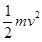where, m = mass of the object, v = velocity of the object(motion)

Question 8. The kinetic energy of an object of mass, m moving with a velocity of 5 m s-1 is 25 J. What will be its kinetic energy when its velocity is doubled? What will be its kinetic energy when its velocity is increased three times?
Solution :
K.E. of the object

=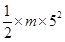m = (25 x 2) /25 = 2 kg

If velocity is doubled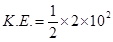= 200/2 = 100 J i.e. K.E. will become four times

If velocity is increased three times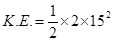= 225 J i.e. K.E. will become nine times

Question 9. What is power?
Solution : Power is the rate of work done. It is denoted by P.

power = work/time

P = W/t

Question 10. Define 1 watt of power.
Solution : If an agent works at the rate of 1 J/s then the power of that agent is 1 watt (where watt is the unit of power).

Question 11. A lamp consumes 1000 J of electrical energy in 10 s. What is its power?
Solution :
P = W/t = 1000J/10s

= 100 W

Question 12. Define average power.
Solution : An agent may not be able to perform same amount of work in a given period of time always. In other words, power of that agent may vary with time. Hence we can take average power in such situations. Average power is defined as average amount of work done by a body per unit time (i.e. total energy consumed divided by total time).

Question 13. Look at the activities listed below. Reason out whether or not work is done in the light of your understanding of the term ‘work’.

• Suma is swimming in a pond.

• A donkey is carrying a load on its back.

• A wind-mill is lifting water from a well.

• A green plant is carrying out photosynthesis.

• An engine is pulling a train.

• Food grains are getting dried in the sun.

• A sailboat is moving due to wind energy.

Solution : Work will be done if a force acts on an object and displacement occurs in the direction of force. According to this explanation work is done in following activities:

• Suma is swimming in a pond.

• A donkey is carrying a load on its back.

• A wind-mill is lifting water from a well.

• An engine is pulling a train.

• A sailboat is moving due to wind energy.

Question 14. An object thrown at a certain angle to the ground moves in a curved path and falls back to the ground. The initial and the final points of the path of the object lie on the same horizontal line. What is the work done by the force of gravity on the object?
Solution : For calculating work done there must be displacement but since in this example vertical displacement is zero (as initial and final points lie on the same horizontal line) so the work done by force of gravity is zero.

Question 15. A battery lights a bulb. Describe the energy changes involved in the process.
Solution : Chemical energy of battery is changed into heat energy and light energy.

Question 16. Certain force acting on a 20 kg mass changes its velocity from y 5 m s-1 to 2 m s-1. Calculate the work done by the force.
Solution :
Work done by the force = change in kinetic energy

= 210 J

Question 17. A mass of 10 kg is at a point A on a table. It is moved to a point B. If the line joining A and B is horizontal, what is the work done on the object by the gravitational force? Explain your answer.
Solution : Since work done on the object by gravitational force depends upon change in the vertical height of the object. Vertical height of the object is not changing as the joining A and B is horizontal at the same height hence the work done is zero.

Question 18. The potential energy of a freely falling object decreases progressively. Does this violate the law of conservation of energy? Why?
Solution : It doesn’t violate the law of conservation of energy because the potential energy of a freely falling object decreases progressively since it keeps changing into kinetic energy of the free falling object therefore the total energy to the object remains conserved.

Question 19. What are the various energy transformations that occur when you are riding a bicycle?
Solution : It is the transformation of our muscular energy into mechanical energy to ride the bicycle.

Question 20. Does the transfer of energy take place when you push a huge rock with all you might and fail to move it? Where is the energy you spend going?
Solution : While we push a huge rock with all our might(power) but fail to move it no energy transfer occurs as cellular energy simply wastes out in muscle contraction and relaxation even heat generation(sweating).

Question 21. A certain household has consumed 250 units of energy during a month. How much energy is this in joules?
Solution :
Energy consumed by a certain household = 250 kWh

since 1 kWh = 3.6 x 106 J

therefore 250 kWh

= 250 x 3.6 x 106

= 9 x 108 J

Question 22. An object of mass 40 kg is raised to a height of 5 m above the ground. What is its potential energy? If the object is allowed to fall, find its kinetic energy when it is half way down.
Solution :
Potential energy

(P) = m x g x h = 40 x 5 x 10

= 2000 J

When the object is half way down the height of the object is = 2.5 m

initial velocity (u) = 0 (thrown from ground/rest)

since, v2 = u2 + 2gh

= 0 + 2 x 10 x 2.5

= 50

Kinetic energy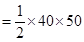= 1000 J

Question 23. What is the work done by the force of gravity on a satellite moving round the earth? Justify your answer.
Solution : The satellite is moving on a round path, displacement in the object is perpendicular to the direction of force.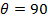.

Therefore, work done is zero.

Question 24. Can there be displacement of an object in the absence of any force acting on it? Think. Discuss this Question with your friends and teacher.
Solution : No.

Question 25. A person holds a bundle of hay over his head for 30 minutes and gets tired. Has he done some work or not? Justify your answer.
Solution : When a person holds a bundle of hay over his head for 30 minutes and gets tired he applies force in upward direction and displacement of bundle of hay is in forward direction which is perpendicular to the direction of force applied therefore displacement is zero

No work done.

Question 26. An electric heater is rated 1500 W. How much energy does it use in 10 hours?
Solution :
Electric heater’s power (p)

= 1500 W

= 1.5 kW

Energy = power x time

= 1.5 KW x 10 h

= 15 kWh

Question 27. Illustrate the law of conservation of energy by discussing the energy changes which occur when we draw a pendulum bob to one side and allow it to oscillate. Why does the bob eventually come to rest? What happens to its energy eventually? Is it a violation of the law of conservation of energy?
Solution : The bob eventually comes to rest due to the frictional force offered by the air and the rigid support holding the thread.
It is not a violation of the law of conservation of energy since mechanical energy can get converted into another form of energy which cannot be utilised for useful work. This loss of energy is called dissipation of energy.

Question 28. An object of mass,m is moving with a constant velocity,v. How much work should be done on the object in order to bring the object to rest?
Solution : In order to bring the object to rest the work done must be equal to the kinetic energy of the moving object

Question 29. Calculate the work required to be done to stop a car of 1500 kg moving at a velocity of 60 km/h?
Solution :
Initial velocity of the car (u)

= 60 km/h

= (60 x 1000) / 60 x 60

= 50 / 3 ms-1

Final velocity (v) = 0 (object has to be stopped)

Initial kinetic energy

= 208333.30 J

Final kinetic energy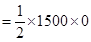= 0

Therefore, work done = change in kinetic energy

= 208333.30 ― 0 = 208333.30 J

Question 30. In each of the following a force,F is acting on an object of mass,m. The direction of displacement is from west to east shown by the longer arrow. Observe the diagrams carefully and state whether the work done by the force is negative, positive or zero.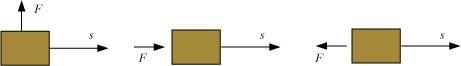Solution :

(i) Since in this diagram displacement is perpendicular to the direction of force, so work done is zero.

(ii) Since in this diagram displacement is in the direction of force, so work done is positive.

(iii) Since in this diagram displacement is in the opposite direction of the force applied hence work done is negative.

Question 31. Soni says that the acceleration in an object could be zero even when several forces are acting on it. Do you agree with her? Why?
Solution : Yes we agree with her statement. Because when many balanced forces act on the object its displacement becomes zero.

Question 32. Find the energy in kW h consumed in 10 hours by four devices of power 500 W each.
Solution :
Since Energy = power x time

= 4 x 500 x 10= 20000 Wh

= 20 kWh

Question 33. A freely falling object eventually stops on reaching the ground. What happens to its kinetic energy?
Solution : A free falling object eventually stops on reaching the ground since on striking the ground its kinetic energy is transmitted to the ground.

## Conclusions for NCERT SOLUTIONS FOR CLASS 9 SCIENCE CHAPTER 11 WORK AND ENERGY

SWC academic staff has developed NCERT answers for this chapter of the ninth grade science curriculum. We have solutions prepared for all the ncert questions of this chapter. The answers, broken down into steps, to all of the questions included in the NCERT textbook’s chapter are provided here. Read this chapter on theory. Be certain that you have read the theory section of this chapter of the NCERT textbook and that you have learnt the formulas for the chapter that you are studying.